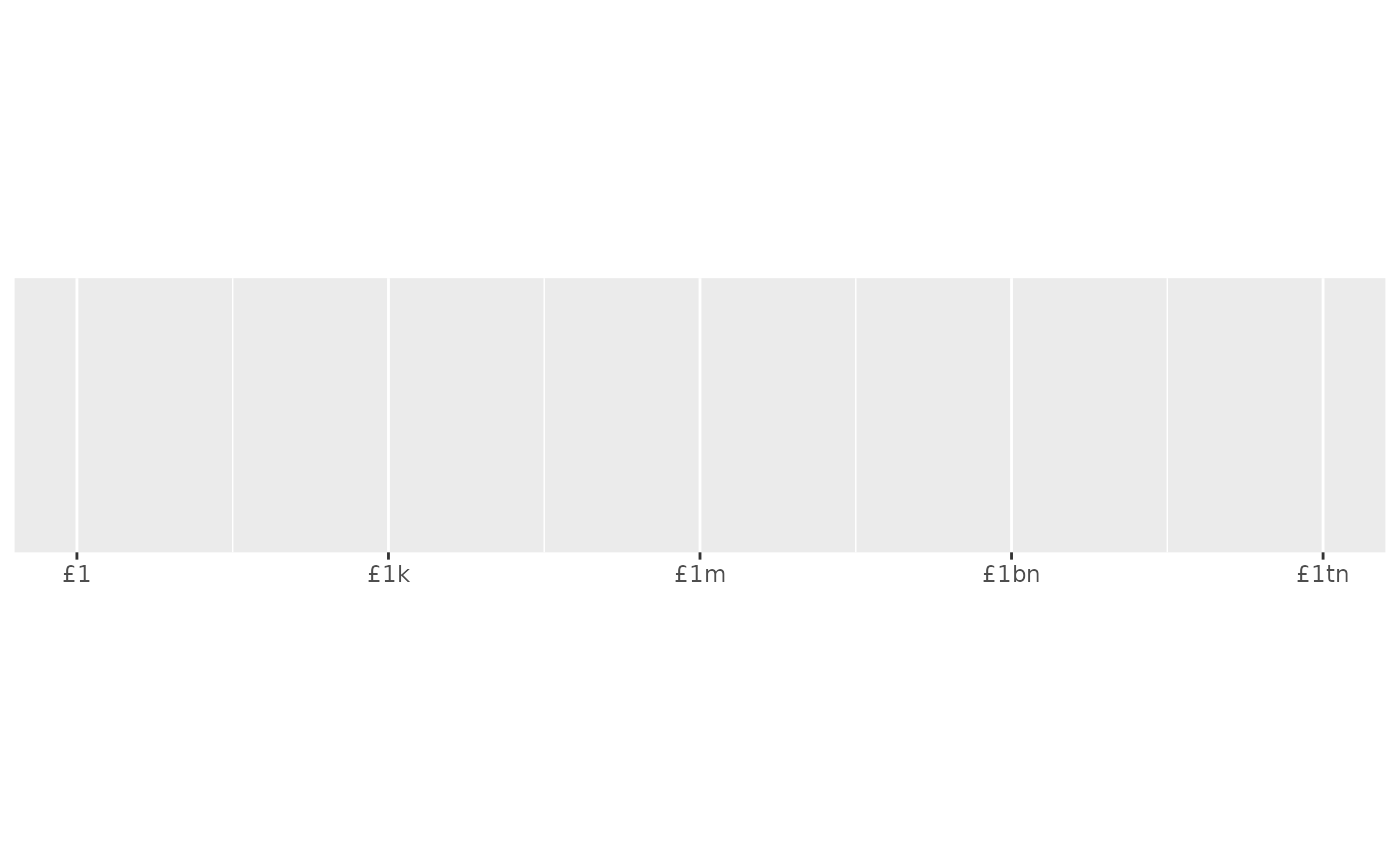Format numbers as currency, rounding values to dollars or cents using a convenient heuristic.

label_dollar(
accuracy = NULL,
scale = 1,
prefix = "$", suffix = "", big.mark = ",", decimal.mark = ".", trim = TRUE, largest_with_cents = 1e+05, negative_parens = FALSE, rescale_large = NULL, ... ) dollar_format( accuracy = NULL, scale = 1, prefix = "$",
suffix = "",
big.mark = ",",
decimal.mark = ".",
trim = TRUE,
largest_with_cents = 1e+05,
negative_parens = FALSE,
rescale_large = NULL,
...
)

dollar(
x,
accuracy = NULL,
scale = 1,
prefix = "\$",
suffix = "",
big.mark = ",",
decimal.mark = ".",
trim = TRUE,
largest_with_cents = 1e+05,
negative_parens = FALSE,
rescale_large = NULL,
...
)

rescale_short_scale()

rescale_long_scale()

## Arguments

accuracy, largest_with_cents Number to round to. If NULL, the default, values will be rounded to the nearest integer, unless any of the values has non-zero fractional component (e.g. cents) and the largest value is less than largest_with_cents which by default is 100,000. A scaling factor: x will be multiplied by scale before formatting. This is useful if the underlying data is very small or very large. Symbols to display before and after value. Character used between every 3 digits to separate thousands. The character to be used to indicate the numeric decimal point. Logical, if FALSE, values are right-justified to a common width (see base::format()). Display negative using parentheses? Named list indicating suffixes given to large values (e.g. thousands, millions, billions, trillions). Name gives suffix, and value specifies the power-of-ten. The two most common scales are provided (rescale_short_scale() and rescale_long_scale()). If NULL, the default, these suffixes aren't used. Other arguments passed on to base::format(). A numeric vector

## Value

All label_() functions return a "labelling" function, i.e. a function that takes a vector x and returns a character vector of length(x) giving a label for each input value.

Labelling functions are designed to be used with the labels argument of ggplot2 scales. The examples demonstrate their use with x scales, but they work similarly for all scales, including those that generate legends rather than axes.

## Old interface

dollar() and format_dollar() are retired; please use label_dollar() instead.

## See also

Other labels for continuous scales: label_bytes(), label_number_auto(), label_number_si(), label_ordinal(), label_parse(), label_percent(), label_pvalue(), label_scientific()

## Examples

demo_continuous(c(0, 1), labels = label_dollar())
#> scale_x_continuous(labels = label_dollar())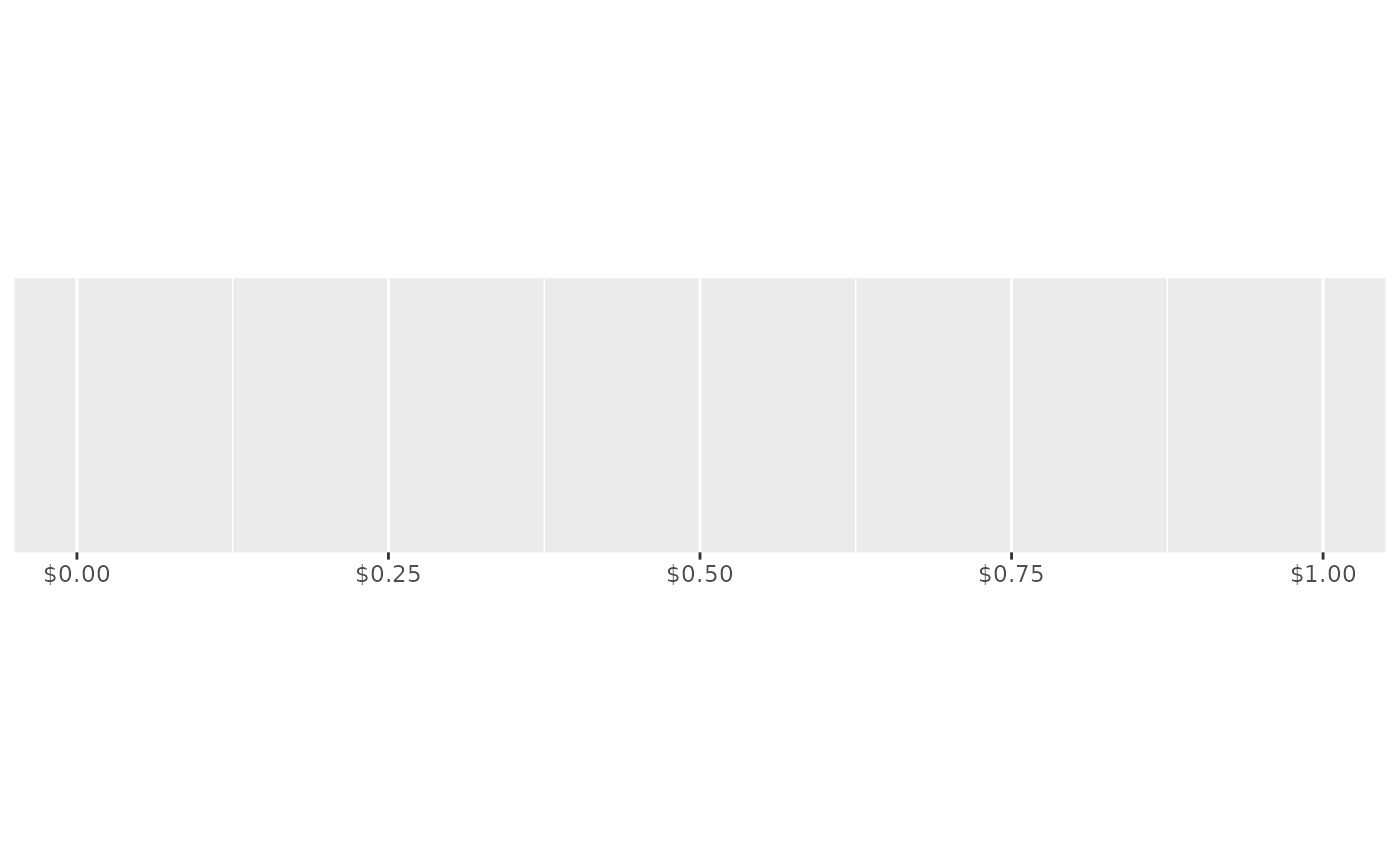demo_continuous(c(1, 100), labels = label_dollar())
#> scale_x_continuous(labels = label_dollar())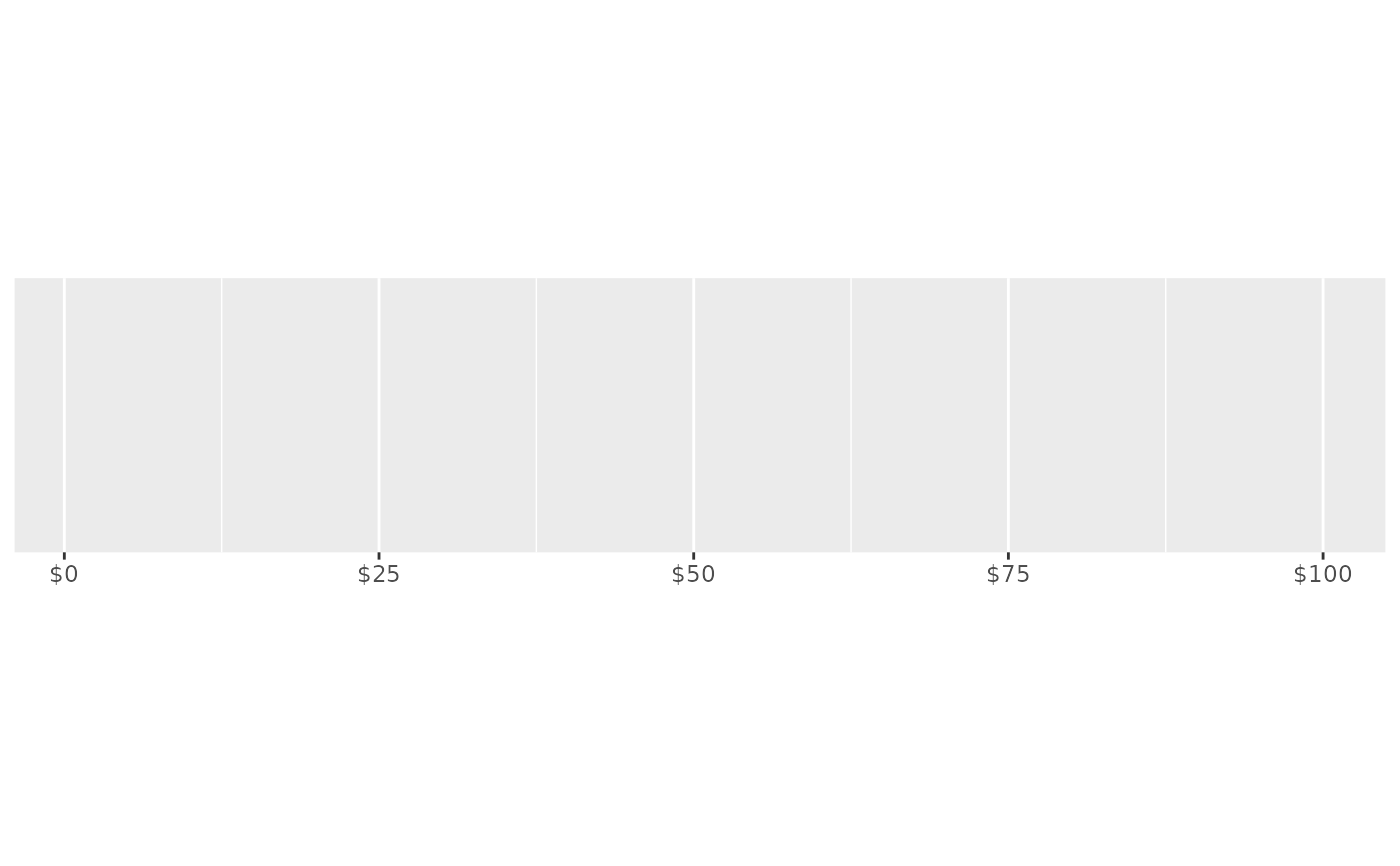# Customise currency display with prefix and suffix
demo_continuous(c(1, 100), labels = label_dollar(prefix = "USD "))
#> scale_x_continuous(labels = label_dollar(prefix = "USD "))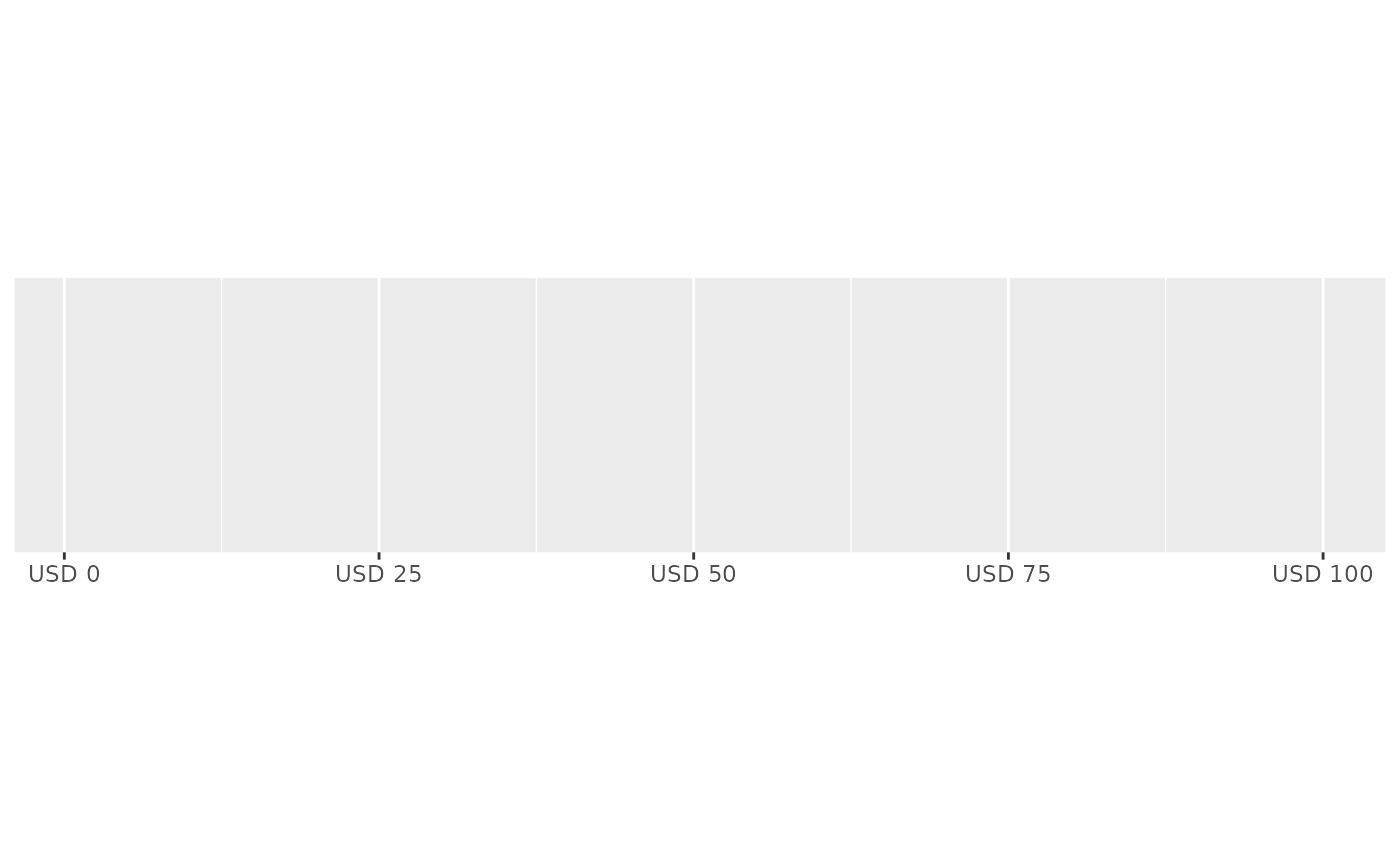euro <- label_dollar(
prefix = "",
suffix = "\u20ac",
big.mark = ".",
decimal.mark = ","
)
demo_continuous(c(1000, 1100), labels = euro)
#> scale_x_continuous(labels = euro)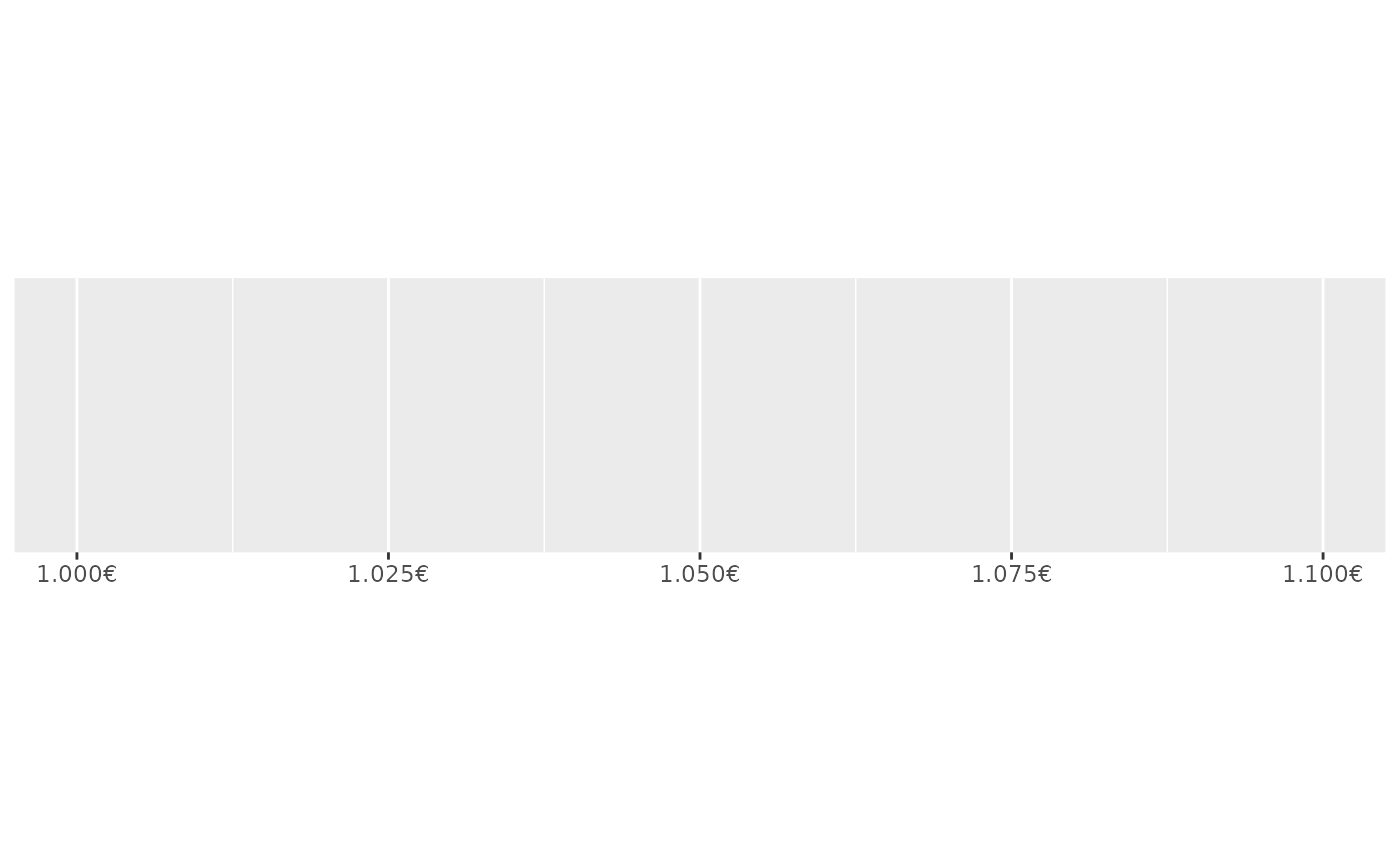# Use negative_parens = TRUE for finance style display
demo_continuous(c(-100, 100), labels = label_dollar(negative_parens = TRUE))
#> scale_x_continuous(labels = label_dollar(negative_parens = TRUE))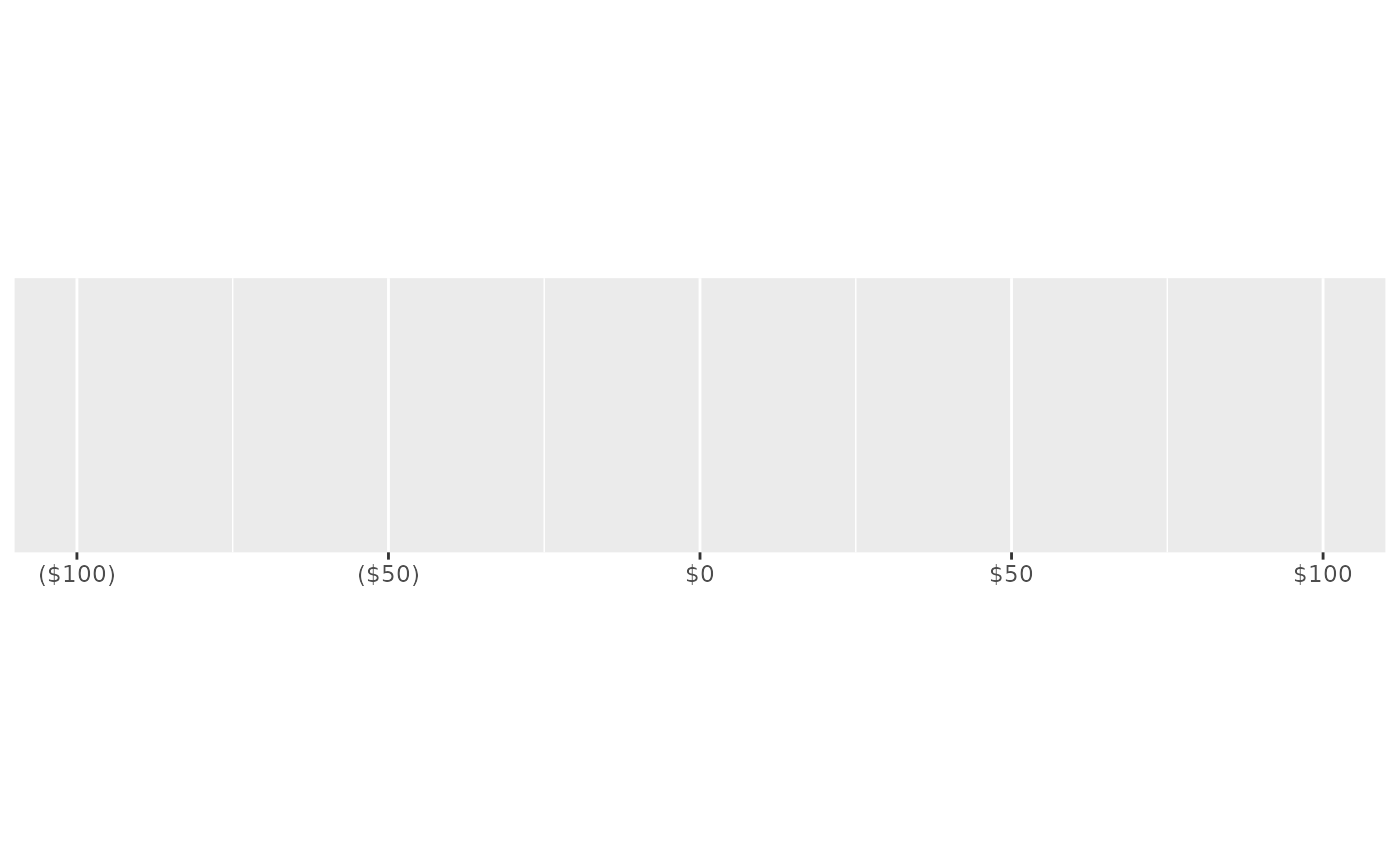# In finance the short scale is most prevalent
dollar <- label_dollar(rescale_large = rescale_short_scale())
demo_log10(c(1, 1e18), breaks = log_breaks(7, 1e3), labels = dollar)
#> scale_x_log10(breaks = log_breaks(7, 1000), labels = dollar)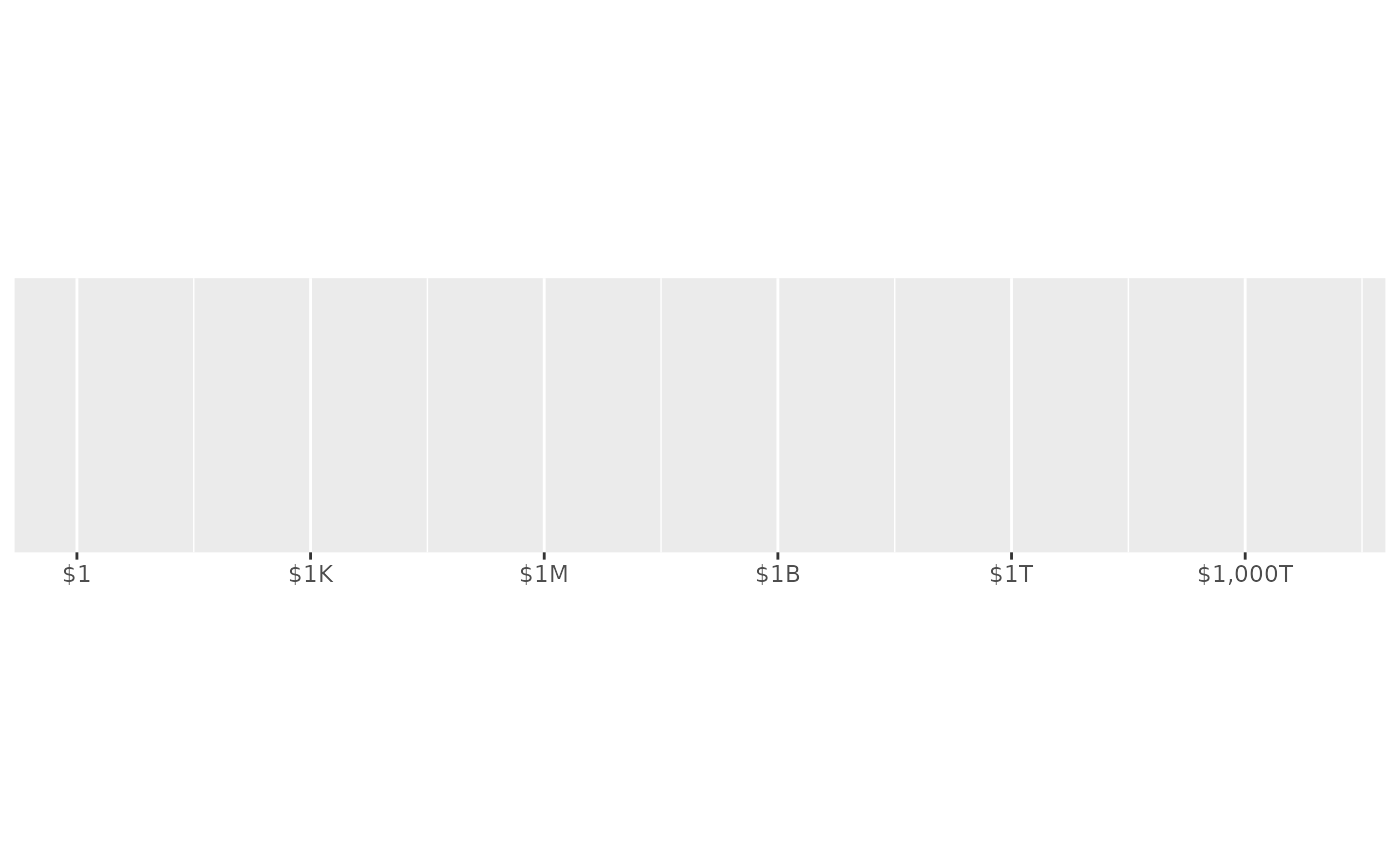# In other contexts the long scale might be used
long <- label_dollar(prefix = "", rescale_large = rescale_long_scale())
demo_log10(c(1, 1e18), breaks = log_breaks(7, 1e3), labels = long)
#> scale_x_log10(breaks = log_breaks(7, 1000), labels = long)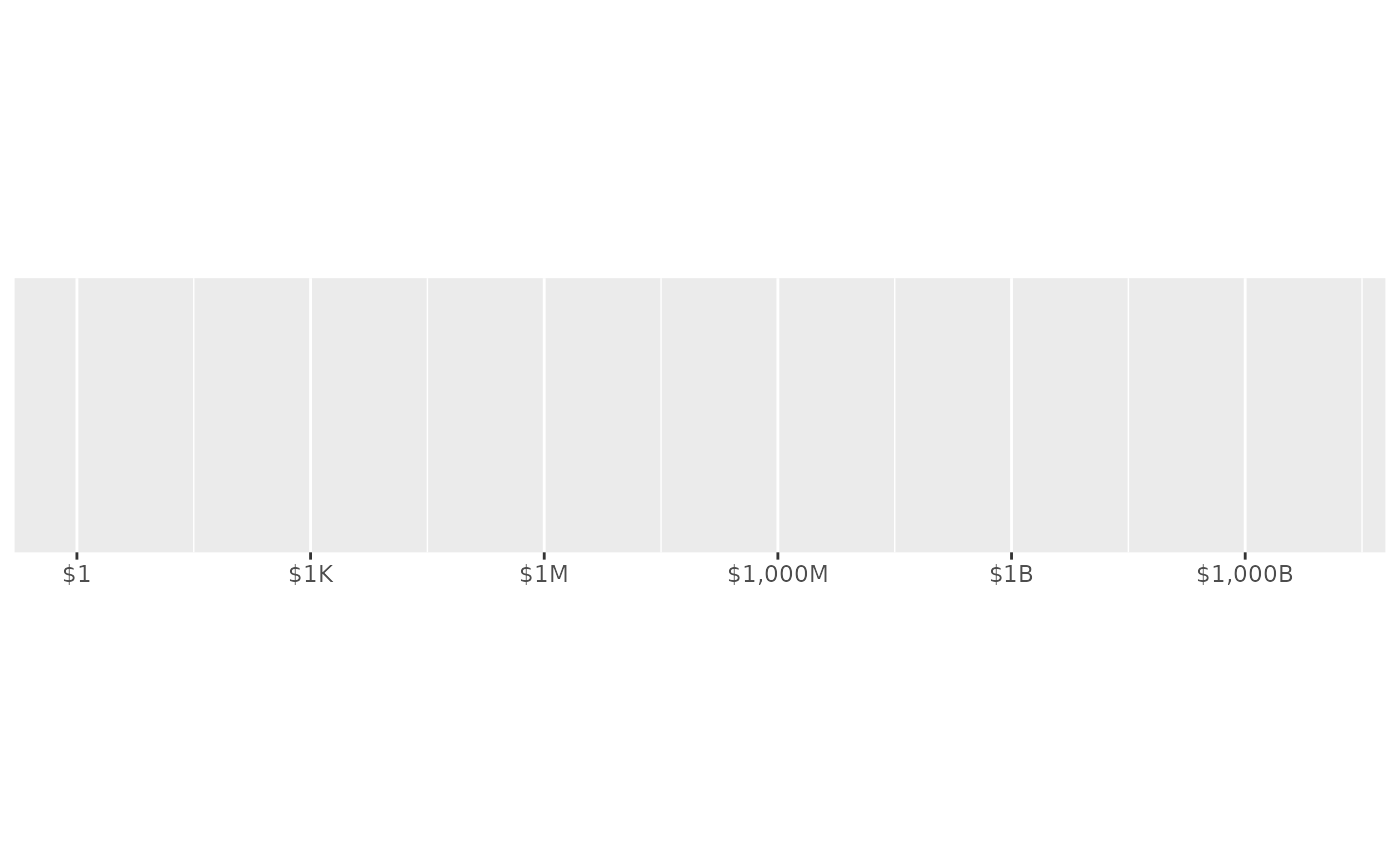# You can also define a custom naming scheme
gbp <- label_dollar(
prefix = "\u00a3",
rescale_large = c(k = 3L, m = 6L, bn = 9L, tn = 12L)
)
demo_log10(c(1, 1e12), breaks = log_breaks(5, 1e3), labels = gbp)
#> scale_x_log10(breaks = log_breaks(5, 1000), labels = gbp)